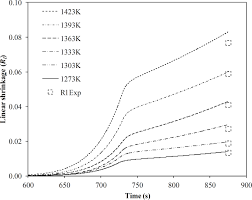## How to Calculate and Solve for Fired Linear Shrinkage | Refractories

The fired linear shrinkage is illustrated by the image below.To compute for fired linear shrinkage, two essential parameters are needed and these parameters are Dried Length (DL) and Fired Length (FL).

The formula for calculating fired linear shrinkage:

FLS = 100(DL – FL/DL)

Where:

FLS = Fired Linear Shrinkage
DL = Dried Length
FL = Fired Length

Given an example;
Find the fired linear shrinkage when the dried length is 32 and the fired length is 12.

This implies that;

DL = Dried Length = 32
FL = Fired Length = 12

FLS = 100(DL – FL/DL)
FLS = 100((32 – 12)/32)
FLS = 100(20/32)
FLS = 100(0.625)
FLS = 62.5

Therefore, the fired linear shrinkage is 62.5%.